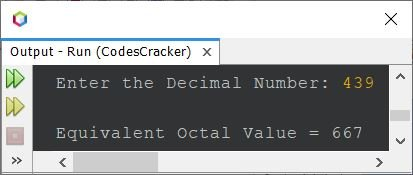# Java Program to Convert Decimal to Octal

This post covers a program in Java that converts a decimal number entered by user at run-time of the program, to its equivalent octal value.

Note - If you're not aware about, how the decimal to octal conversion takes place, then refer to Decimal to Octal Conversion.

## Decimal to Octal Conversion in Java

The question is, write a Java program to convert decimal number to octal. The decimal number must be received by user at run-time. The program given below is its answer:

```import java.util.Scanner;

public class CodesCracker
{
public static void main(String[] args)
{
int decimal, rem, i=0;
int[] octal = new int;

Scanner scan = new Scanner(System.in);

System.out.print("Enter the Decimal Number: ");
decimal = scan.nextInt();

while(decimal != 0)
{
rem = decimal%8;
octal[i] = rem;
i++;
decimal = decimal/8;
}

System.out.print("\nEquivalent Octal Value = ");
for(i=(i-1); i>=0; i--)
System.out.print(octal[i]);
}
}```

The sample run of above program with user input 439 as decimal number to convert and prints its equivalent octal value, is shown in the snapshot given below:In above program, the following block of code:

```rem = decimal%8;
octal[i] = rem;
i++;
decimal = decimal/8;```

can also be replaced with the block of code, given below:

```octal[i++] = decimal%8;
decimal /= 8;```

#### Same Program in Other Languages

Java Online Test

« Previous Program Next Program »